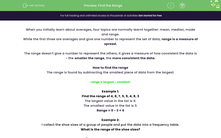# Find the Range

In this worksheet, students will find the range from both lists and tables.Key stage:  KS 4

Year:  GCSE

GCSE Subjects:   Maths

GCSE Boards:   AQA, Eduqas, Pearson Edexcel, OCR,

Curriculum topic:   Statistics

Curriculum subtopic:   Statistics Analysing Data

Difficulty level:#### Worksheet Overview

When you initially learn about averages, four topics are normally learnt together: mean, median, mode and range.

While the first three are averages and give one number to represent the set of data, range is a measure of spread.

The range doesn't give a number to represent the others, it gives a measure of how consistent the data is - the smaller the range, the more consistent the data.

How to find the range

The range is found by subtracting the smallest piece of data from the largest:

range = largest - smallest

Example 1:

Find the range of 4, 8, 7, 5, 9, 4, 8, 3

The largest value in the list is 9.

The smallest value in the list is 3.

Range = 9 - 3 = 6

Example 2:

I collect the shoe sizes of a group of people and put the data into a frequency table.

What is the range of the shoe sizes?

 Shoe size 3 4 5 6 7 8 9 10 11 12 Number of people 5 7 9 14 21 28 16 6 5 1

We need to be careful here, the largest number that appears in the table is 28, but this is a frequency, not a shoe size. We never use frequency when we are finding the range.

The largest shoe size that appears is 12.

The smallest shoe size that appears is 3.

The range of shoe sizes is 12 - 3 = 9

Let's move on to the questions now.

### What is EdPlace?

We're your National Curriculum aligned online education content provider helping each child succeed in English, maths and science from year 1 to GCSE. With an EdPlace account you’ll be able to track and measure progress, helping each child achieve their best. We build confidence and attainment by personalising each child’s learning at a level that suits them.

Get started••••